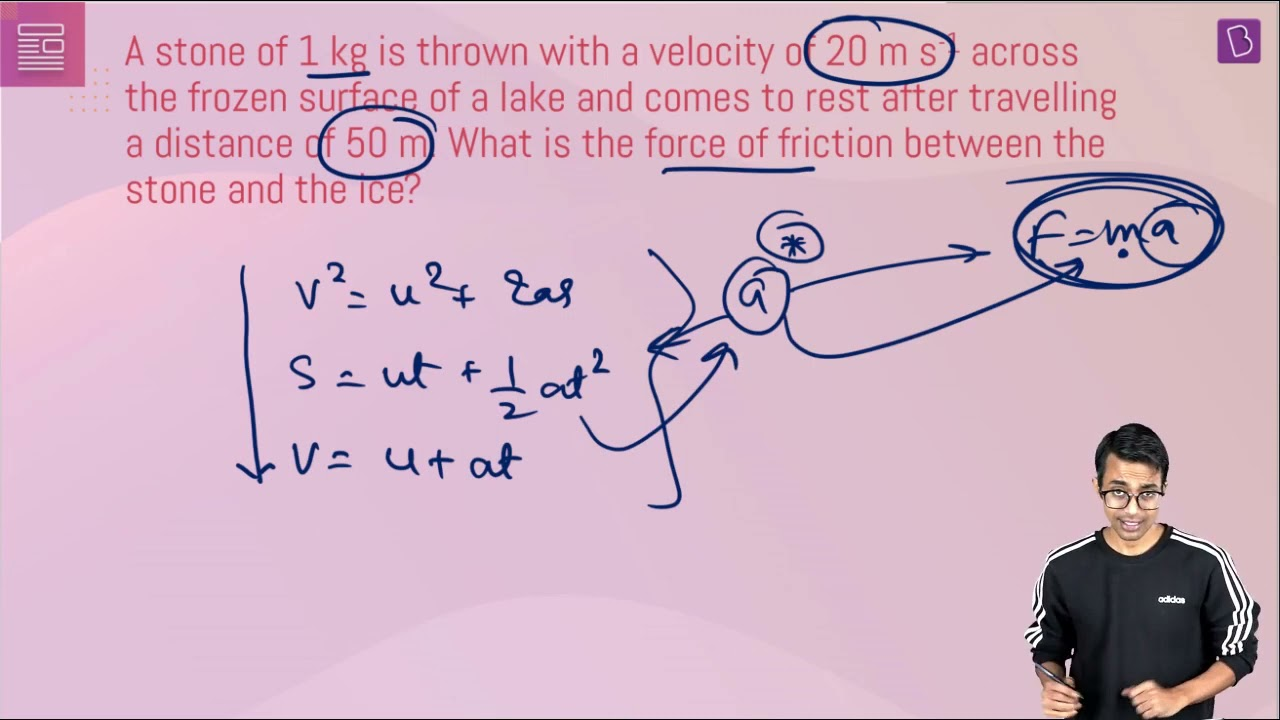# A stone of 1 kg is thrown with a velocity of 20 m s-1 across the frozen surface of a lake and comes to rest after travelling a distance of 50 m. What is the force of friction between the stone and the ice?Given

The initial velocity of the stone, u= 20 m/s

The final velocity of the stone, v= 0

Distance covered by the stone, s= 50 m

Find out

The force of friction between the stone and the ice

Solution

We know the third equation of motion
v² = u² + 2as

Substituting the known values in the above equation we get,

0² = (20)² + 2(a)(50)

-400 = 100a

a = -400/100  =  -4m/s² (retardation)

We know that

F = m×a

Substituting above obtained value of a = -4 in F = m x a we get,

F = 1 × (-4) = -4N

4 (61)(210)(19)

#### Choose An Option That Best Describes Your Problem

Thank you. Your Feedback will Help us Serve you better.

#### 1 Comment

1. Gauri gupta

I loved how it is so detailed and explained very nicely